## Tamilnadu Samacheer Kalvi 9th Maths Solutions Chapter 2 Real Numbers Ex 2.6

Ex 2.6 Class 9 Question 1.
Simplify the following using addition and subtraction properties of surds:
(i) 5$$\sqrt { 3 }$$ + 18$$\sqrt { 3 }$$ – 2$$\sqrt{3}$$
(ii) 4$$\sqrt [ 3 ]{ 5 }$$ + 2$$\sqrt [ 2 ]{ 5 }$$ – 3$$\sqrt [ 3 ]{ 5 }$$
(iii) 3$$\sqrt { 75 }$$ + 5$$\sqrt { 48 }$$ – $$\sqrt { 243 }$$
(iv) 5$$\sqrt [ 3 ]{ 40 }$$ + 2$$\sqrt [ 3 ]{ 625 }$$ – 3$$\sqrt [ 3 ]{ 320 }$$
Solution:
(i) 5$$\sqrt { 3 }$$ + 18$$\sqrt { 3 }$$ – 2$$\sqrt { 3 }$$ = (5 + 18 – 2)$$\sqrt { 3 }$$ = 21$$\sqrt { 3 }$$
(ii) 4$$\sqrt [ 3 ]{ 5 }$$ + 2$$\sqrt [ 2 ]{ 5 }$$ – 3$$\sqrt [ 3 ]{ 5 }$$ = (4 + 2 – 3)$$\sqrt [ 3 ]{ 5 }$$ = 3$$\sqrt [ 3 ]{ 5 }$$
(iii) 3$$\sqrt { 75 }$$ + 5$$\sqrt { 48 }$$ – $$\sqrt { 243 }$$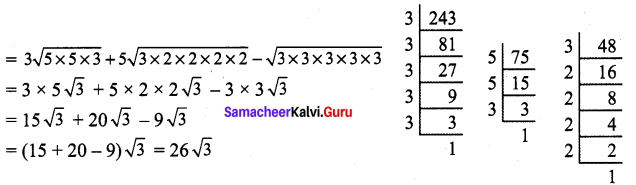(iv) 5$$\sqrt [ 3 ]{ 40 }$$ + 2$$\sqrt [ 3 ]{ 625 }$$ – 3$$\sqrt [ 3 ]{ 320 }$$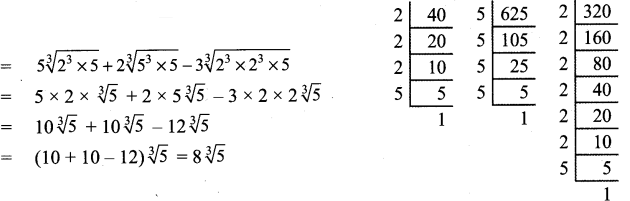2.6 9th Class Math Question 2.
Simplify the following using multiplication and division properties of surds :
(i) $$\sqrt{3} \times \sqrt{5} \times \sqrt{2}$$
(ii) $$\sqrt { 35 }$$ ÷ $$\sqrt { 7 }$$
(iii) $$\sqrt{27} \times \sqrt{8} \times \sqrt{125}$$
(iv) (7$$\sqrt { a }$$ – 5$$\sqrt { b }$$)(7$$\sqrt { a }$$ + 5$$\sqrt { b }$$)
(v) $$[\sqrt{\frac{225}{729}}-\sqrt{\frac{25}{144}}] \div \sqrt{\frac{16}{81}}$$
Solution: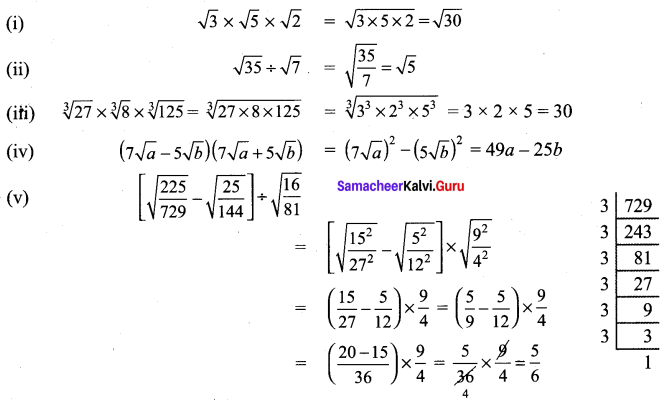Maths Class 9 Exercise 2.6 Solutions Question 3.
If $$\sqrt { 7 }$$ =1.414, $$\sqrt { 3 }$$ = 1.732, $$\sqrt { 5 }$$ = 2.236, $$\sqrt { 10 }$$ = 3.162, then find the values of the following correct to 3 places of decimals.
(i) $$\sqrt{40}-\sqrt{20}$$
(ii) $$\sqrt{300}+\sqrt{90}-\sqrt{8}$$
Solution: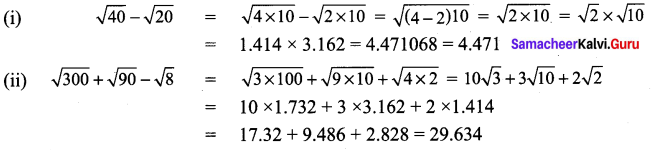9th Class Maths Exercise 2.6 Solution Question 4.
Arrange surds in descending order :
(i) $$\sqrt{5}, \sqrt{4}, \sqrt{3}$$
(ii) $$\sqrt{\sqrt{5}}, \sqrt{\sqrt{7}}, \sqrt{\sqrt{3}}$$
Solution:
(i) $$\sqrt{5}, \sqrt{4}, \sqrt{3}$$
5$$\frac { 1 }{ 3 }$$
∴ The order of the surds $$\sqrt{5}, \sqrt{4}, \sqrt{3}$$ are 3, 9, 6.
4$$\frac { 1 }{ 9 }$$
3$$\frac { 1 }{ 6 }$$ l.c.m of 3, 9, 6 is 18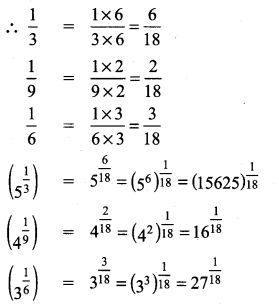∴ The descending order of $$\sqrt{5}, \sqrt{4}, \sqrt{3}$$ is $$(15625)^{\frac{1}{18}}>(27)^ {\frac{1}{18}} >16^{\frac{1}{18}} \text { i.e. } \sqrt{5}>\sqrt{3}>\sqrt{4}$$

(ii) $$\sqrt{\sqrt{5}}, \sqrt{\sqrt{7}}, \sqrt{\sqrt{3}}$$
The order of the surds $$\sqrt{\sqrt{5}}, \sqrt{\sqrt{7}}, \sqrt{\sqrt{3}}$$ are 6, 12, 4
l.c.m of 6, 12, 4 is 12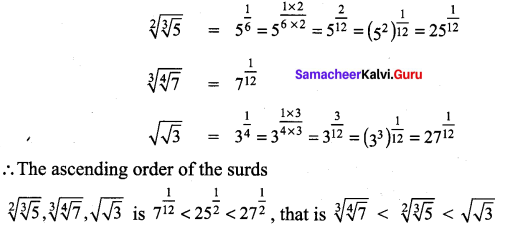Class 9 Maths Ex 2.6 Solution Question 5.
Can you get a pure surd when you find
(i) the sum of two surds
(ii) the difference of two surds
(iii) the product of two surds
(iv) the quotient of two surds
Justify each answer with an example.
Solution: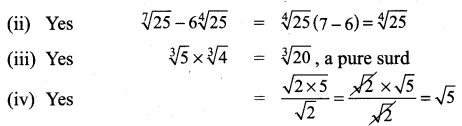Exercise 2.6 Class 9 Maths Question 6.
Can you get a rational number when you compute
(i) the sum of two surds
(ii) the difference of two surds
(iii) the product of two surds
(iv) the quotient of two surds
Justify each answer with an example.
Solution:
(i) Yes (5 – $$\sqrt{3}$$) + (5 + $$\sqrt{3}$$) = 10, a rational number
(ii) Yes $$(5+\sqrt{7})-(-6+\sqrt{7})=11$$, a rational number
(iii) Yes (5 + $$\sqrt{3}$$ ) (5 – $$\sqrt{3}$$ ) = 25 – 3 = 22, a rational number
(iv) Yes $$\frac{5 \sqrt{3}}{\sqrt{3}}=5$$ ,a rational number